# Two Machine Learning Models for Mobile Phone Battery Discharge Rate Prediction Based on Usage Patterns

## Article information

Journal of Information Processing Systems. 2016;12(3):436-454
Publication date (electronic) : 2016 September 30
doi : https://doi.org/10.3745/JIPS.03.0048
*Dept. of Computer Engineering, Faculty of Engineering, Kasetsart University, Bangkok, Thailand (fengcnc@ku.ac.th)
**Faculty of Business Administration, Rajamangala University of Technology Rattanakosin, Klai Kangwon Palace campus, Hua Hin, Thailand (maimai_mp@hotmail.com)
Corresponding Author: Chantana Chantrapornchai (fengcnc@ku.ac.th)
Received 2014 November 14; Revised 2015 August 10; Accepted 2016 April 06.

## Abstract

This research presents the battery discharge rate models for the energy consumption of mobile phone batteries based on machine learning by taking into account three usage patterns of the phone: the standby state, video playing, and web browsing. We present the experimental design methodology for collecting data, preprocessing, model construction, and parameter selections. The data is collected based on the HTC One X hardware platform. We considered various setting factors, such as Bluetooth, brightness, 3G, GPS, Wi-Fi, and Sync. The battery levels for each possible state vector were measured, and then we constructed the battery prediction model using different regression functions based on the collected data. The accuracy of the constructed models using the multi-layer perceptron (MLP) and the support vector machine (SVM) were compared using varying kernel functions. Various parameters for MLP and SVM were considered. The measurement of prediction efficiency was done by the mean absolute error (MAE) and the root mean squared error (RMSE). The experiments showed that the MLP with linear regression performs well overall, while the SVM with the polynomial kernel function based on the linear regression gives a low MAE and RMSE. As a result, we were able to demonstrate how to apply the derived model to predict the remaining battery charge.

## 1. Introduction

Nowadays, everyone relies on mobile phones on a daily basis. Smartphones have appeared with the advancement of technology, and are called as such as they equipped with more functions to allow users to watch videos, listen to music, take photos, browse the Internet, navigate, etc. However, running these applications requires different levels of power consumption. Normally, a phone battery has a very limited capacity based on its make. Knowing the power usage of the applications can help conserve battery power so that it can last as long as possible before it has to be recharged.

Phone hardware is composed of different kinds of CPU, memory, etc. All of which consume power differently. The power consumed by various applications differs because each application requires different resources and uses different instructions in order to function properly. The inputs for each application affect the power usage for that application. For instance, the different levels of LCD brightness result in different power consumption. To figure out the power usage model of each application, one must explore factors for the specific application. It may be complex to define mathematically such exact formula.

This paper presents the prediction models for the battery discharge rate of mobile phones considering each usage pattern. The challenge of this work relies on the data preprocessing phase. First, what are the usage factors that affect the power consumption? How are we going to collect the power consumptions for each varying factor? There are many possible applications that the user may run and each of them can use different inputs. The software tools used for measuring battery consumption are rare, and they mostly depend on the mobile phone platform and operating systems. Getting the exact values of battery consumption requires the usage of hardware .

With the various characteristics of each resource usages we studied, the model can predict the energy consumption for a particular application. Once it is known how much energy they use, the rest of the energy in the phone battery can be managed efficiently. At the end of this paper, we present an example of applying the model to predict the remaining battery for future use.

## 2. Background

In this work, we applied the machine learning technique to construct the prediction model. Typical machine learning is classified as supervised learning and unsupervised learning. Supervised learning is based on the desired output. The data set used for training provides the correct output, and the input connecting to the correct output is created. Unsupervised learning is done without a supervisor, meaning the output is not checked. The network arranges its structure in accordance with the characteristics of the data.

The approaches we considered were the artificial neural network and the support vector machine (SVM). The artificial neural network emulates the nervous system in the human brain. Each process in the neural network receives the input and computes it, and the output is generated. The output of the neural network is then compared to the desired output. If the obtained output has an error, the error is used to adjust the weight of each input.

The structure of the network is composed of a group of nodes that connect to one another in each layer, which includes the input layer, hidden layers, and output layer. The neural network can have a multilayer structure called a multilayer perceptron (MLP). With the advantages of multilayer, MLP is well suited for modeling a complicated task. This network trains itself through supervised learning by using a method called back propagation. The process of back propagation is comprised of two parts: the forward pass and backward pass.

The MLP consists of layers of nodes formed as a directed graph, where each node is a neuron with an activation function. MLP is an extension of the standard linear perceptron. To construct this type of network, one needs to figure out the proper neural architecture, such as the number of hidden layers. Furthermore, the activation function types are selected, and the learning algorithm is selected. The learning algorithm of the multilayer perceptron is more complex since the weights of all hidden layers need to be updated. The weight update depends on the error calculation, the learning rate, and the momentum rate. The momentum and the learning rate have values that are less than 1. The momentum is the scaling factor for escaping from the local minima. The learning rate is selected in such a way that the weights converge fast enough, depending on the number of epoch.

SVM is a supervised learning method used for classifying data. Typically, it separates data into two categories. In general, several SVM classifiers can be combined to perform the multi-classifier. In theory, the goal is to find the good decision boundary, which separates the two classes as much as possible. A kernel function is used to measure the similarity between any two items. Various kernel functions, including the polynomial function, sigmoid function, radius basic function, Gaussian function, etc., can be considered .

A smartphone battery is usually made from lithium-ion or a lithium polymer because they are very low profile, lightweight and safe . The lifetime of the battery is expected to be 300–500 charging cycles. The full charging time is about 2–3 hours. Current smartphones are equipped with many functions and supports. The supports for 3G and 4G are very important for fast data transfer. However, using these networks quickly runs down the phone battery. Apart from 3G and 4G, there are other wireless technologies, such as Wi-Fi, GPS, Bluetooth, etc. When all of these are enabled, they can consume more than 25% of the total power dissipation in some platforms . Also, current smartphones use a touch-screen display, which is built using LCD. A large LCD consumes more power. The smartphone’s CPU can be a dual core or quad core processor. Using high frequency CPU will result in more power consumption.

## 3. Previous Work

Several researchers found that user-related behavior affects mobile phone power consumption. They presented a power consumption estimation model using various approaches. Typical mobile phones, such as Nokia, HTC, Google Nexus, etc., were used in the experiments. The user’s usage patterns, which affect the lifetime of the battery, were also explored.

Carroll conducted an analysis of power consumption in a smartphone . She presented a detailed analysis of the power consumption of a mobile phone called Openmoko Neo Freerunner. The consumed energy, which was based on several usages, such as audio, video, SMS, web, and phone applications, was measured. It was found that each pattern uses different levels of power. Two other phones, HTC and Google Nexus One, were used as a testing platform. In addition, a power model of a Freerunner device was developed. The analysis of the energy usage and battery lifetime with many usage patterns, as well as the energy impact of dynamic voltage and frequency scaling of the device’s application processor were all considered.

Zhao et al.  proposed an approach for predicting the lifetime of the battery for the HTC G1 smartphone by using system contexts influencing the lifetime of the battery. For the statistical method, the multiple linear regression model was used to predict the lifetime of the battery. An experiment of playing a video and setting up the system context’s values for five variables was conducted. Then, the energy consumption for each variable derived from multiple linear regression was developed.

Korhonen  proposed an approach for predicting battery lifetime using a Nokia device. This work was aimed at developing a model for calculating the remaining battery life as well as battery consumption for each application. Five variables were considered for the selected applications, and energy consumption was measured within a certain time period. All values for each variable were set while taking into account external variables, such as the volume level of an application call, Wi-Fi being on/off, brightness level of the monitor, etc.

Kang et al. [7,8] introduced an approach to predict lifetime of the battery by taking into account different usage patterns. First, the experiments were carried out based on the context that influences the lifetime of the battery. Next, data collection was done at a particular time of the day, such as at noon, in the afternoon, in the evening, or during a holiday. Twenty sample populations were used in this research. The data collected was analyzed for creating a formula to predict battery lifetime on the basis of usage patterns and the period of time.

Banerjee et al.  presented the Llama system to manage battery energy for a mobile phone. User behavior was studied carefully in the aspect of battery usage and recharging behavior. Llama learned from the user behavior and adjusted the service to preserve the battery as much as possible.

Panigrahi et al.  presented a model for mathematically estimating the lifetime of the battery. Rakhmatov et al.  proposed a model for the battery lifetime for a pocket computer. They considered the case of constant load and variable load. The test was done on a sample pocket computer based on Strong ARMSA-1100. They compared the model estimation with the results from Peukert’s Law.

Aliev et al.  considered the battery charging process. They developed a way to extract rules to speed up the charging process. Balasubramanian et al.  focused on the network applications for mobile phones. TailEnder was proposed to reduce energy consumption. It is a scheduling algorithm that manages networking in the mobile phone while providing good download performance and consuming less energy. Donohoo  used machine-learning techniques to learn user’s usage patterns on a mobile device. He focused on 3G, Wi-Fi, and GPS. The algorithm adaptively adjusts the application and control parameters to conserve energy.

This work differs from many existing one as follows: compared to , we also considered GPS, Sync and Bluetooth states, while  only considered the Wi-Fi, GPS, and Bluetooth states. A multiple linear regression model was used in  for the prediction model, and we used the machine learning approach to create the regression model. The presented framework can support both linear and polynomial regression. For , they considered building an algorithm that adjusts the setting to minimize energy consumption.

## 4. Methodology

To find a battery prediction model based on various system settings and application usages, we studied the characteristics from previous works in [4,7,8,14] in detail. Furthermore, some up-to-date factors, such as Bluetooth, brightness, 3G, GPS, Wi-Fi, and Sync were added. Machine learning was applied to build the regression model for prediction. SVM and MLP with different kernel functions were used to build the prediction models. The experimental methodology is explained in this paper and the effectiveness of each possible derived model was tested.

We collected 480 sets of different levels of battery samples for our experiments, as described below. The selected applications were used as benchmarks varying their usages. The battery level was inspected and the time intervals were recorded. The hardware used was HTC One V with an Android operating system.

### 4.1 Data Collection and Analysis

The system characteristics examined are presented as in Table 1. Our approach can be used to build both linear regression and polynomial regression for prediction models. The possible values for each attribute are discretized, as described in Table 1.

Next, we analyzed the relationships between these factors using multiple regression analysis (i.e., using Stepwise) in SPSS software. These seven factors were used and one dependent variable was considered. Three application usages are explained below.

1. Standby mode: CPU, brightness, 3G, GPS, Wi-Fi, and Bluetooth are the most dependent variables (about 14.4%), with S.E.est =0.17 with a statistical significant value less than 0.05.

2. Video: Brightness, 3G, Bluetooth, Wi-Fi, CPU, Sync, and GPS are the most dependent variables (about 25.7%), with S.E.est=0.63 with a statistical value that is significantly less than 0.05.

3. Web browser: 3G, Bluetooth, brightness, Wi-Fi, CPU, Sync, and GPS are the most dependent variables (about 23.3%), with S.E.est=0.59 with a statistical value that is significantly less than 0.05.

Tables 24 show the sample set of status vectors applied for each application. The total data set generated was 320, 480, and 480 for the standby mode, the video application, and the web browser application.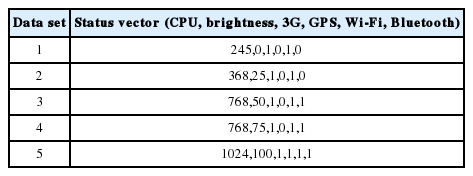Status vector example for standby (CPU, brightness, 3G, GPS, Wi-Fi, Bluetooth)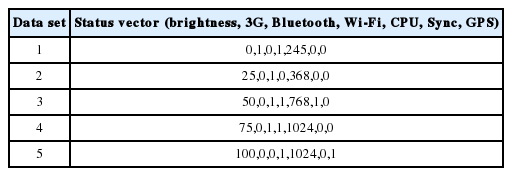Status vector example for video (brightness, 3G, Bluetooth, Wi-Fi, CPU, Sync, GPS)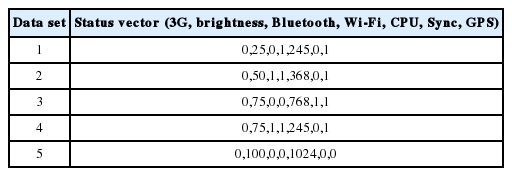Status vector example for web browser (3G, brightness, Bluetooth, Wi-Fi, CPU, Sync, GPS)

The applications used to setup the mobile phone according to the status vectors in Tables 24 were SetCPU and Select brightness level, as shown in Fig. 1(a) and (b). After that, the battery level of the mobile phone was measured with Battery Doctor, as shown in Fig. 1(c). The values were measured every 5 minutes within 1 hour.

After all of the values of battery levels were gathered, a chart of the battery’s levels was made, the equation for estimating battery levels using linear regression is shown in Fig. 2. This was done in the same manner for the three applications of standby, video, and web browser. In Fig. 3(a), we present a sample plot of battery levels (for 3G). We used the sampling data every 5 minutes for each state. The battery discharge rate was based on the coefficients calculated and on the changes in battery level over time. The slope of the graph shows this. The dashed line shows the estimation of the discharge rate using the linear function. Fig. 3(b) presents the prediction using the 3rd order polynomial function. It has been noted that it may be close to the real data sampled. However, using the polynomial order 3 requires three coefficients. To predict the discharge rate, three outputs (for three coefficients, x3, x2, and x) rather than one output (for one coefficient x) are needed.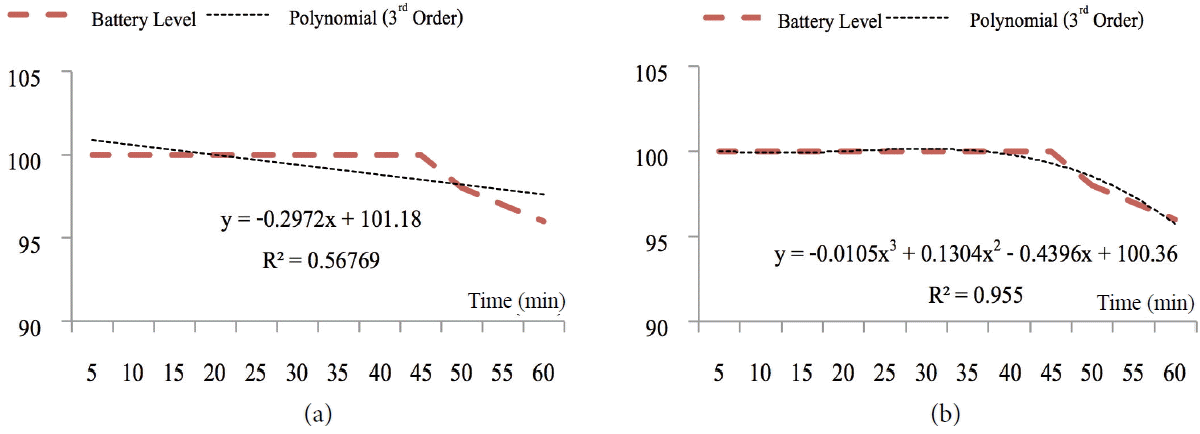Battery discharge rate (a) linear regression (b) polynomial.

### 4.2 Creation of the Model for Predicting the Discharge Rate of the Battery Rate

Machine learning was used to create the prediction model. The two techniques are MLP and SVM. The three models were created for the three application models with different state vectors. The data set was separated into 320 sets for standby, 480 for web browser, and 480 for video applications. The experiments were based on supervised learning, where 10-fold cross validation was used.

For each model, we performed 10-fold cross validation. The data sets were divided into 10 groups. The first group was assigned as a testing set and the remaining nine were used as training sets. The process was carried out by rotating these groups 10 times, and the testing set was changed until completion. Then, the accuracy of the model was measured based on the root mean squared error (RMSE). In Eq. (1)n is the total data, zi is the real value, and yi is the predicted value.

(1) RMSE=i=1n(zi-yi)2n

The mean absolute error (MAE) was measured as shown in Eq. (2), where n is the total data, zi is the real value, and yi is the predicted value.

(2) MAE=1ni=1n|zi-yi|

These values were compared for both approaches for the linear regression and polynomial regression prediction models. For SVM, we compared the models using the three kernel functions of linear, polynomial, and radius basis.

## 5. Numerical Results

This section presents the accuracy results of prediction models for MLP and SVM with three applications: standby, video, and web browser. For video, we ran the sample video ‘30 Kumlang Jaew’ for 1 hour while collecting the battery value. For the web browser application, we explored the new web page topic (http://en.wikipedia.org/wiki/Artificial_neural_network) for 5 minutes for 1 hour.

The models were constructed to predict the linear coefficient and 3rd order polynomial coefficients. MAE and RSME values were compared for each coefficient. For the 3rd order polynomial function, there were 3 outputs since there were three coefficients. We are only reporting the average MAE and RSME of all of the coefficients in this case.

### 5.1 MLP Models

The MLP model from the linear regression equation and the polynomial regression equation were developed. Listed below are the common parameters that affect the construction of a learning model.

1. Learning rate: This is the value between [0,1]. A lower learning rate will slow down but it may still provide high precision, while a high learning rate may not be good if the data is overly distributed.

2. Momentum: This is the value between [0,1]. Less distributed data may yield more momentum to provide smooth learning, while more distributed data may require a lower momentum to oscillate around the data set.

3. The number of hidden layers reflects the linearity of data. A zero hidden layer means linearly separable data.

Good training parameters lead to the fewer errors and greater precision. From our preliminary experiments we found the possible parameters, which are shown in Table 5, for a 120 test cases.

In the following section, we only displayed the best parameter for each application where the MAE and RMSE were the smallest.

#### MLP with linear regression

The accuracy results of the standby, video, and web browser applications are shown in Table 6.

For all 120 test cases for each application, the best result yielded the following parameters for standby: Learning rate=0.01, Momentum=0.3, Hidden layer=3, and N=1,000, where we got MAE =0.0493 and RMSE=0.078. For the video application, we got MAE=0.2543 and RMSE=0.341, which is the same as for the standby mode. For the web browser application, the best results were from the following parameters: Learning rate=0.01, Momentum=0.3, Hidden layer=3 and N=500, where MAE=0.206 and RMSE=0.2771.

#### MLP with polynomial regression

Table 7 shows the best parameter values for MLP for polynomial regression. In this case, we considered three coefficients in the order 3, 2 and 1 (x3, x2, and x), which corresponds to Output 1, Output 2, and Output 3, respectively. Thus, for higher polynomial degrees, the network needs more outputs.

### 5.2 SVM Model

For SVM, the parameters that may affect the accuracy are C, gamma, and degree, as well as the types of kernels. C is the parameter for the cost function. A bigger C tends to cause an over-fitting of the data, while a C that is too small may cause under-fitting. Gamma is the parameter for the kernel, and degree is the degree of the polynomial function. We considered three types of SVM kernels: linear, polynomial, and radius basic function (RBF). We also considered linear regression, which has one output, and polynomial regression, which has three similar outputs.

#### SVM with linear regression

For the SVM with a linear kernel, we varied the cost (C) from 1 to 11. The results for SVM with a linear kernel function were not affected by the cost value. All cost values result in the same MAE and RMSE for all standby, video, and web browser applications. The standby cases yielded the same MAE and RMSE, which were MAE=0.0737 and RMSE=0.1002. The video case yielded the same MAE and RMSE, which were MAE=0.2651 and RMSE=0.3603. All web browser cases yielded the same MAE and RMSE, which were MAE=0.2603 and RMSE=0.3316.

For the SVM with a polynomial kernel, the degrees were varied in [1..4], gamma in [0.0, 0.2, 0.4, 0.6], and C in [1..11]. There were 44 test cases in total, and the best result parameters are shown in Table 8 for each application.

Table 8 shows that the good parameters for standby are degree=3, gamma=0.2, and C=1, which yielded MAE=0.0633 and RMSE=0.0842. For the video application, the best model used degree=3 and gamma=0.4, C=1, which resulted in MAE=0.2428 and RMSE=0.338. For the web browser application, the best model used degree=2 and gamma=0.6, C=1, which yielded MAE=0.198 and RMSE=0.269. For this kernel function, the best configuration varied depending on the type of application, but all of them still used C=1.

For the SVM with an RBF kernel (shown in Table 9), the best parameter configurations for the standby mode were gamma=0.2 and C=1, where MAE=0.621 and RMSE=0.0853. For the video application, the parameters were gamma=0.4 and C=1, where MAE=0.2413 and RMSE=0.3243. For the web browse application, the parameters were gamma=0.0 and C=1, where MAE=0.2114 and RMSE=0.283. All the applications used C=1.

#### SVM with the polynomial regression

Table 10 summarizes the best results for SVM with the polynomial regression by applications for linear kernel for each output.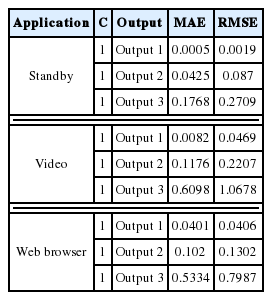Best MAE and RMSE for SVM with the linear kernel for polynomial regression

Table 10 shows the MAE and RMSE values for each usage and for each output. The MAE value was the highest for the case of Output 3 for each application, and Output 1 had the lowest MAE. This is also true for Tables 11 and 12, which both use a different kernel function.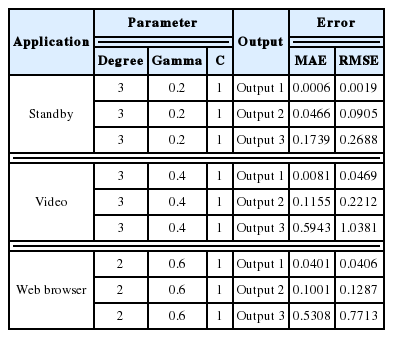Best MAE and RMSE for SVM with the polynomial kernel for polynomial regression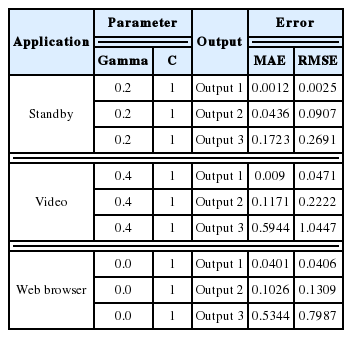Best MAE and RMSE for SVM with the RBF kernel for polynomial regression

The MAE for each output increased for the polynomial kernel and the RBF kernel for each output and for each application in the same manner in Table 12.

### 5.3 Comparison Results

Fig. 4 compares the MAE for each SVM kernel for each output for the polynomial regression. It is seen that for Output 1 that the linear kernel and polynomial kernel performed best for standby and video applications. For Output 2, the linear kernel, polynomial kernel, and polynomial kernel performed best for standby, video, and web browser applications, respectively. For Output 3, the RBF kernel, polynomial kernel, and polynomial kernel gave the smallest MAE for Standby, video, and web browser applications, respectively. It is noted that the MAE for Output 1 was very small compared to the rest.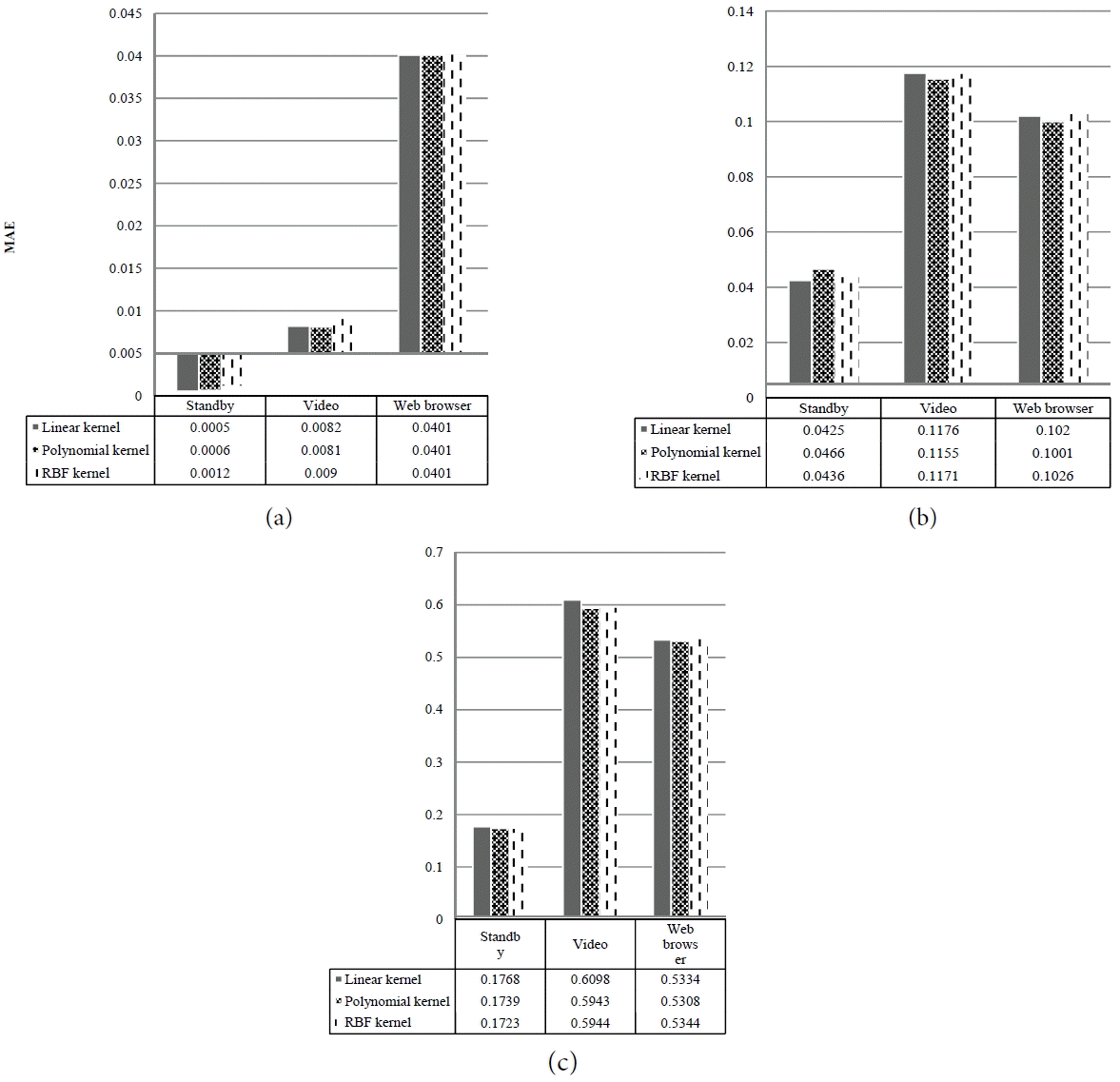Comparison of MAE for polynomial regression prediction for each output: (a) Output 1, (b) Output 2, and (c) Output 3 for SVM kernels.

Fig. 5 compares the MAE for each function and for each application for MLP. Also, the MAE values for each application, MLP, each SVM kernel function, and each regression model are summarized using the average value. It is seen that for MLP that the linear regression consistently predicts the regression coefficients accurately. One reason for this is that the polynomial regression has three outputs where some of the outputs (e.g., Output 3) have large MAE values. Therefore, it is very likely that using the average value degrades the overall MAE values for the polynomial regression model.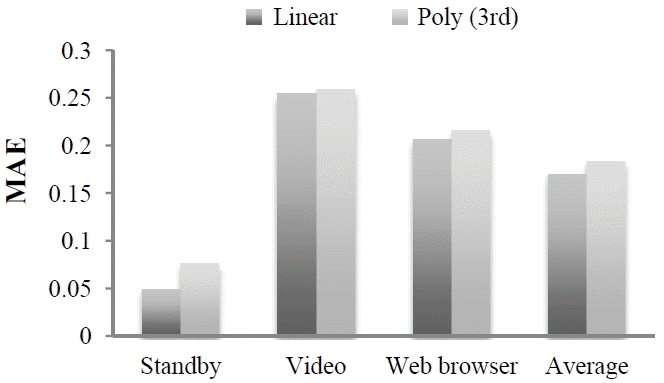Comparison of MAE for 2 regression types (overall by applications).

For SVM, the model with polynomial regression had better accuracy in some applications (from Fig. 6). Looking at the MAE for each kind of SVM, it is seen that the MAE is less for the linear regression prediction for standby. For the video application, polynomial regression prediction performs well for the linear kernel function. For the web browser application, linear regression prediction performs well for the polynomial kernel function.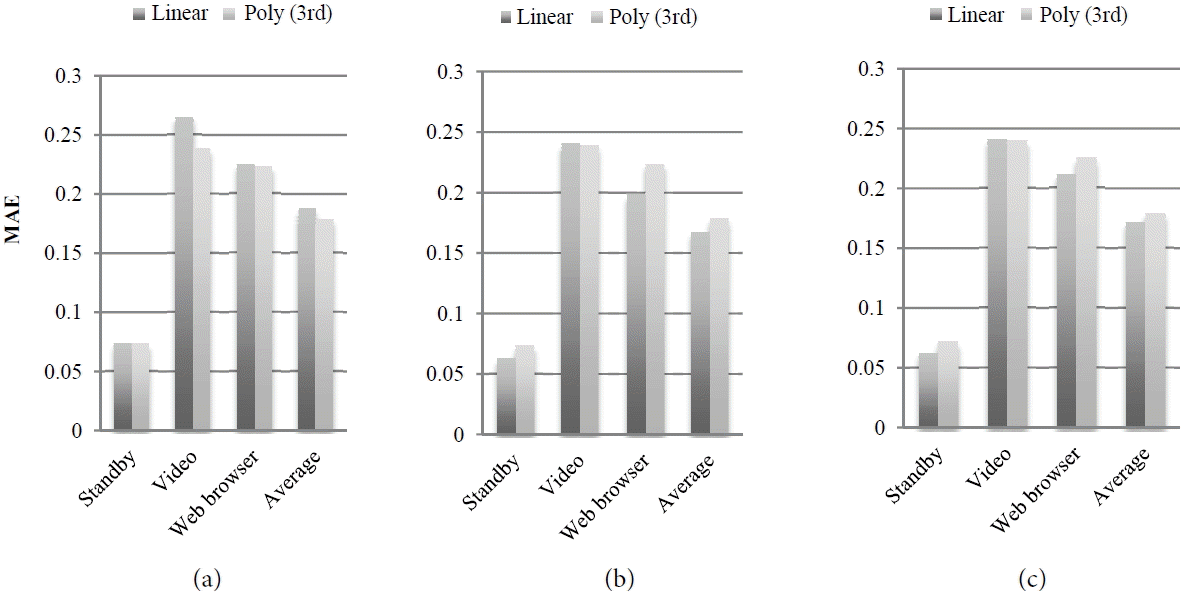Comparison of MAE for each function and each application for (a) SVM (linear kernel), (b) SVM (polynomial), and (c) SVM (RBF).

In Fig. 7, we show the average MAE by application. It was found that linear regression prediction is better overall. Also, the model for the standby mode provides better precision than other applications. The SVM with a linear kernel better predicts the coefficient of the linear regression function for the video application. The SVM with a linear kernel can predict the linear regression coefficient for the web browser application better. As shown in Fig. 8, the model that uses linear regression has fewer errors in every case. The SVM with a polynomial kernel has fewer errors than that the MLP.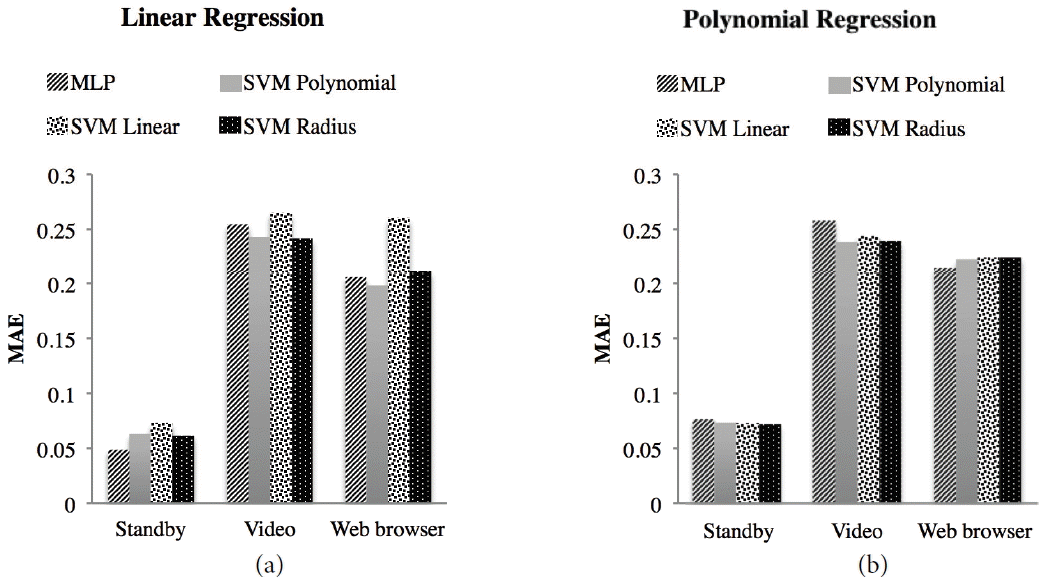Comparison of MAE for MLP and all SVMs: (a) linear regression and (b) polynomial regression.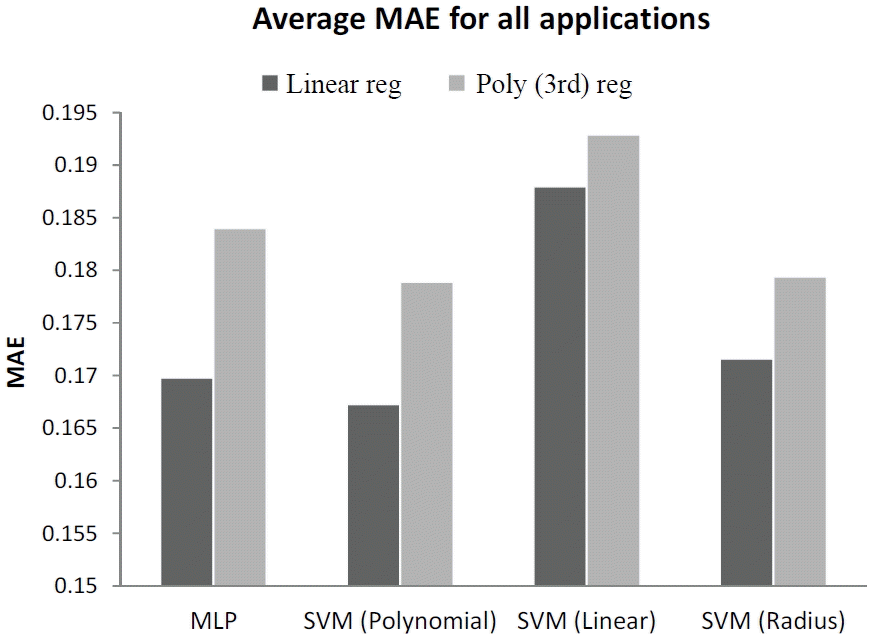Average MAE comparison for MLP and SVM with different regression functions.

## 6. Applications of the Models

What we obtained from the model was the proper regression models and coefficients. We then inspected the values of the battery discharge rate for each status vector, as shown in Table 13.

From the status vector setting, we set the phone in the state The amount of energy released within an hour was measured. This is shown in Eq. (3):

(3) Hour left=Remaining energy (mAh)Battery discharge rate (%mA)

Fig. 9 is the battery discharge rate, which was 0.24%. This graph shows the setting, CPU=10.24, Wi-Fi=1, 3G=1, Bluetooth=1, brightness=100, and GPS=1. We calculated the remaining hours as follows: The battery capacity was 1,500 mA (for 100%) where the discharge rate was 0.24% per minute. That is 3.6 mA for every 5 minutes or about 55.70 mA within an hour. Thus, for a 1,500 mA battery, the phone can be used in the standby mode about 27 hours. Table 14 presents an example of the calculation for different status vectors in the standby mode.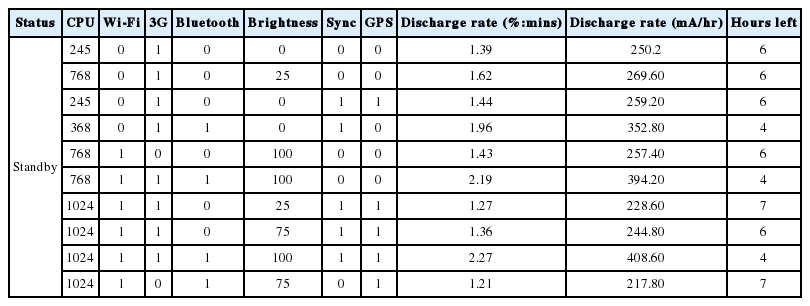Sample calculation for different status vectors (standby)

Similarly, we were able to calculate the discharge rate at mA/hr. for the video application shown in Table 15 using the obtained the discharge rate model shown in Fig. 10 and for the web browser shown in Table 16 with the discharge rate shown in Fig. 11. In Fig. 10, the discharge rate was 2.23%. This graph is for the setting: CPU=1,024, Wi-Fi=0, 3G=1, Bluetooth=1, brightness=75, Sync=1, and GPS=1. Thus, for every 5 minutes, the application consumed 33.45 mA. For one hour, it consumed 401.4 mA. For the whole battery capacity of 1,500 mA, the application can run for up to 4 hours.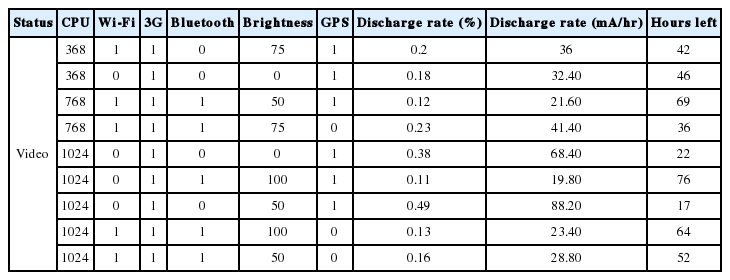Sample calculation for different status vectors (video)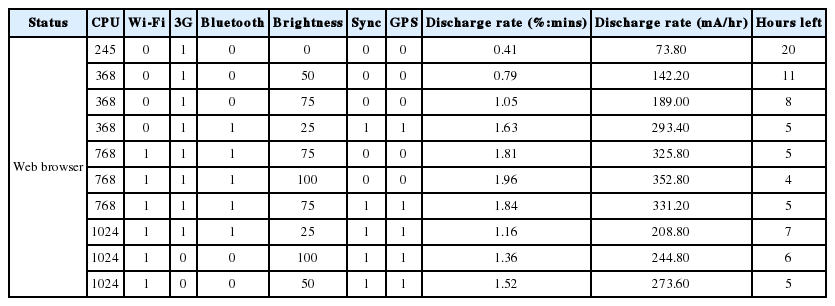Sample calculation for different status vectors (web browser)

For Fig. 11, the plot was obtained for the following setting: CPU=245, Wi-Fi=1, 3G=1, Bluetooth=1, brightness=0, Sync=1, and GPS=1. The discharge rate was 0.89%, which is about 13.35 mA for every 5 minutes. Thus, in 1 hour, the application consumed 160.2 mA. Then, for the whole capacity of the battery of 1,500 mA, we were able to run the application for 9 hours.

With this optimal configuration that we obtained, we compared the overall device discovery time for the existing binary search scheme and the proposed partition-based scheme, as shown in Fig. 8. From this figure, we can see that the proposed scheme gives a much smaller device discovery time than the existing scheme does. Moreover, the performance gaps between the existing and proposed schemes become larger as the number of devices increases. This is because the proposed scheme can reduce the attempts made for device discovery by dividing all devices into several partitions and can also minimize the possibility of multiple responses (collisions) from the devices, as compared to the existing binary search scheme.

## 7. Conclusions

This work presented the framework to create a battery usage model. We used this framework to study seven factors: CPU utilization, brightness, wireless state, Bluetooth, 3G, GPS, and Sync. Different settings for these factors were considered for measuring the resulting battery. The HTC One V was used for data collection. States were used to determine the battery usage for the standby mode, video playing, and web browser applications. The battery usage was determined for each state. From the data collected, we were able to determine the models for predict battery consumption.

We compared the effectiveness of two machine learning models for predicting mobile phone battery usage considering linear and polynomial (3rd order) regression. The two models used were the MLP and SVM. To find effectiveness, we compared using RMSE and MAE for all of the cases. The smaller the value is, then a better model can be used for prediction.

From the results, the prediction model implies that using linear regression has the RMSE and MAE less than using polynomial regression. The SVM with a polynomial kernel has a smaller error value than that of the SVM with linear and RBF kernels. It also has fewer errors than the MLP as well. As the whole, the prediction model with the SVM using a polynomial kernel can predict for the linear regression model with the smallest MAE=6.64% and RMSE=8.99%.

There are some limitations in this research in terms of difficulty of collecting battery level from the mobile phone’s battery. Other variables need to be controlled not to affect the set variables such as location to collect the data Also, there are some limitations caused by the battery itself, such as its quality getting worse due to the experiment being conducted many times. The last issue is to set the factor values, which did not always go as desired.

To improve this work, other factors could be integrated and other characteristics or applications should be taken into account, such as text messaging, listening to music, or talking on the phone, for creating models. The framework can be adapted to test the new hardware and considering other factors simultaneously. Once the discharge rate is known, the automatic adjustments of brightness level, Bluetooth, Sync, 3G, etc., can be done to reduce battery consumption so that it can last longer.

## References

1. Vallina-Rodriguez N, Crowcroft J. Energy management techniques in modern mobile handsets. IEEE Communications Surveys & Tutorials 15(1):179–198. 2013;
2. Murphy KP. Machine Learning: A Probabilistic Perspective Cambridge, MA: MIT Press; 2012.
3. Donohoo BK. Machine learning techniques for energy optimization in mobile embedded systems. M.S. thesis Colorado State University; Fort Collins, CO: 2012.
4. Carroll A, Heiser G. An analysis of power consumption in a smartphone. In : Proceedings of USENIX Annual Technical Conference. Boston, MA; 2010.
5. Zhao X, Guo Y, Feng Q, Chen X. A system context-aware approach for battery lifetime prediction in smart phones. In : Proceedings of the 2011 ACM Symposium on Applied Computing. TaiChung, Taiwan; 2011. p. 641–646.
6. Korhonen K. Predicting mobile device battery life. M.S. thesis Aalto University; Espoo, Finland: 2011.
7. Kang JM, Park CK, Seo SS, Choi MJ, Hong JWK. User-centric prediction for battery lifetime of mobile devices. Challenges for Next Generation Network Operations and Service Management Heidelberg: Springer; 2008. p. 531–534.
8. Kang JM, Seo SS, Hong JWK. Personalized battery lifetime prediction for mobile devices based on usage patterns. Journal of Computing Science and Engineering 5(4):338–345. 2011;
9. Banerjee N, Rahmati A, Corner MD, Rollins S, Zhong L. Users and batteries: interactions and adaptive energy management in mobile systems. UbiComp 2007: Ubiquitous Computing Heidelberg: Springer; 2007. p. 217–234.
10. Panigrahi D, Chiasserini CF, Dey S, Rao R, Raghunathan A, Lahiri K. Battery life estimation of mobile embedded systems. In : Proceedings of 14th International Conference on VLSI Design. Bangalore, India; 2001. p. 57–63.
11. Rakhmatov D, Vrudhula S, Wallach DA. A model for battery lifetime analysis for organizing applications on a pocket computer. IEEE Transactions on Very Large Scale Integration (VLSI) Systems 11(6):1019–1030. 2003;
12. Aliev RA, Aliev RR, Guirimov B, Uyar K. Dynamic data mining technique for rules extraction in a process of battery charging. Applied Soft Computing 8(3):1252–1258. 2008;
13. Balasubramanian N, Balasubramanian A, Venkataramani A. Energy consumption in mobile phones: a measurement study and implications for network applications. In : Proceedings of the 9th ACM SIGCOMM Conference on Internet Measurement Conference. Chicago, IL; 2009. p. 280–293.
14. Shye A, Scholbrock B, Memik G. Into the wild: studying real user activity patterns to guide power optimizations for mobile architectures. In : Proceedings of the 42nd Annual IEEE/ACM International Symposium on Microarchitecture. New York, NY; 2009. p. 168–178.

## Biography

Chantana Chantrapornchai http://orcid.org/0000-0002-8699-5736She obtained her Bachelor degree (Computer Science) from Thammasat University of Thailand in 1991. She graduated from Northeastern University at Boston, College of Computer Science, in 1993 and University of Notre Dame, Department of Computer Science and Engineering, in 1999, for her Master and Ph.D. degrees respectively. Currently, she is an associated professor of Dept. of Computer Engineering, Faculty of Engineering, Kasetsart University, Thailand. Her research interests include: parallel computing, big data processing, semantic web, computer architecture and fuzzy logic.

Paingruthai NusawatShe received M.S. degree from Dept. of Computing, Silpakorn University, Nakorn Pathom, Thailand, in 2014. Her current research interests include data mining, and information system. She is currently a lecturer in a faculty of Business Administration at Rajamangala University of Technology Rattanakosin, Klai Kangwon Palace campus.

## Article information Continued

### Table 1

Factors and details

Attributes Description Example
CPU utilization (CPU) The ratio between the idle time to the total of an interval [245–1024] [245,368…]
Screen brightness (brt) Range from [0–100] in mobile phone [0,25,50…]
Wireless state (Wi-Fi) Disable or Enable [0,1] [0,1]
Bluetooth (bt) Disable or Enable [0,1] [0,1]
3rd generation mobile telecommunications (3G) Disable or Enable [0,1] [0,1]
Global positioning system (GPS) Disable or Enable [0,1] [0,1]
Synchronize (Sync) Disable or Enable [0,1] [0,1]

### Table 2

Status vector example for standby (CPU, brightness, 3G, GPS, Wi-Fi, Bluetooth)

Data set Status vector (CPU, brightness, 3G, GPS, Wi-Fi, Bluetooth)
1 245,0,1,0,1,0
2 368,25,1,0,1,0
3 768,50,1,0,1,1
4 768,75,1,0,1,1
5 1024,100,1,1,1,1

### Table 3

Status vector example for video (brightness, 3G, Bluetooth, Wi-Fi, CPU, Sync, GPS)

Data set Status vector (brightness, 3G, Bluetooth, Wi-Fi, CPU, Sync, GPS)
1 0,1,0,1,245,0,0
2 25,0,1,0,368,0,0
3 50,0,1,1,768,1,0
4 75,0,1,1,1024,0,0
5 100,0,0,1,1024,0,1

### Table 4

Status vector example for web browser (3G, brightness, Bluetooth, Wi-Fi, CPU, Sync, GPS)

Data set Status vector (3G, brightness, Bluetooth, Wi-Fi, CPU, Sync, GPS)
1 0,25,0,1,245,0,1
2 0,50,1,1,368,0,1
3 0,75,0,0,768,1,1
4 0,75,1,1,245,0,1
5 0,100,0,0,1024,0,0

### Table 5

MLP parameter setting variation

 Learning rate [0.1, 0.05, 0.025, 0.01] Momentum [0.3, 0.2, 0.1, 0.05, 0.01] Hidden layer [3, 4, 5] Training time [500, 1000]

### Table 6

MLP with the linear regression (standby)

Application Parameter Error
Learning rate Momentum Hidden layer N MAE RMSE
Standby 0.01 0.3 3 1,000 0.0493 0.078
Video 0.01 0.3 3 1,000 0.2543 0.341
Web browser 0.01 0.01 3 500 0.206 0.2771

### Table 7

MLP with polynomial regression (standby)

Application Parameter Error

Output value Learning rate Momentum Hidden layer N MAE RMSE
Standby Output 1 0.01 0.05 3 500 0.0007 0.001
Output 2 0.01 0.05 3 500 0.0473 0.086
Output 3 0.01 0.05 3 500 0.1824 0.2669

Video Output 1 0.01 0.01 3 500 0.0113 0.0476
Output 2 0.01 0.01 3 500 0.1222 0.2234
Output 3 0.01 0.01 3 500 0.6451 1.0689

Web browse Output 1 0.025 0.2 4 500 0.0051 0.0077
Output 2 0.025 0.3 3 500 0.0984 0.1269
Output 3 0.01 0.01 4 500 0.5461 0.7796

### Table 8

SVM with polynomial kernel

Application Parameter Error
Degree Gamma C MAE RMSE
Standby 3 0.2 1 0.0633 0.0842
Video 3 0.4 1 0.2428 0.3380
Web browser 2 0.4 1 0.1982 0.2689

### Table 9

SVM with radius basic function kernel

Application Parameter Error
C Gamma MAE RMSE
Standby 1 0.2 0.0621 0.0853
Video 1 0.4 0.2413 0.3243
Web browser 1 0.0 0.2114 0.283

### Table 10

Best MAE and RMSE for SVM with the linear kernel for polynomial regression

Application C Output MAE RMSE
Standby 1 Output 1 0.0005 0.0019
1 Output 2 0.0425 0.087
1 Output 3 0.1768 0.2709

Video 1 Output 1 0.0082 0.0469
1 Output 2 0.1176 0.2207
1 Output 3 0.6098 1.0678

Web browser 1 Output 1 0.0401 0.0406
1 Output 2 0.102 0.1302
1 Output 3 0.5334 0.7987

### Table 11

Best MAE and RMSE for SVM with the polynomial kernel for polynomial regression

Application Parameter Output Error

Degree Gamma C MAE RMSE
Standby 3 0.2 1 Output 1 0.0006 0.0019
3 0.2 1 Output 2 0.0466 0.0905
3 0.2 1 Output 3 0.1739 0.2688

Video 3 0.4 1 Output 1 0.0081 0.0469
3 0.4 1 Output 2 0.1155 0.2212
3 0.4 1 Output 3 0.5943 1.0381

Web browser 2 0.6 1 Output 1 0.0401 0.0406
2 0.6 1 Output 2 0.1001 0.1287
2 0.6 1 Output 3 0.5308 0.7713

### Table 12

Best MAE and RMSE for SVM with the RBF kernel for polynomial regression

Application Parameter Output Error

Gamma C MAE RMSE
Standby 0.2 1 Output 1 0.0012 0.0025
0.2 1 Output 2 0.0436 0.0907
0.2 1 Output 3 0.1723 0.2691

Video 0.4 1 Output 1 0.009 0.0471
0.4 1 Output 2 0.1171 0.2222
0.4 1 Output 3 0.5944 1.0447

Web browser 0.0 1 Output 1 0.0401 0.0406
0.0 1 Output 2 0.1026 0.1309
0.0 1 Output 3 0.5344 0.7987

### Table 13

Sample status vectors and discharge rate

Application CPU Wi-Fi 3G Bluetooth Brightness Sync GPS Battery discharge rate (%)
Standby 1024 1 1 1 100 - 1 0.24
Video 1024 0 1 1 75 1 1 2.23
Web browser 245 1 1 1 0 1 1 0.89

### Table 14

Sample calculation for different status vectors (standby)

Status CPU Wi-Fi 3G Bluetooth Brightness Sync GPS Discharge rate (%:mins) Discharge rate (mA/hr) Hours left
Standby 245 0 1 0 0 0 0 1.39 250.2 6
768 0 1 0 25 0 0 1.62 269.60 6
245 0 1 0 0 1 1 1.44 259.20 6
368 0 1 1 0 1 0 1.96 352.80 4
768 1 0 0 100 0 0 1.43 257.40 6
768 1 1 1 100 0 0 2.19 394.20 4
1024 1 1 0 25 1 1 1.27 228.60 7
1024 1 1 0 75 1 1 1.36 244.80 6
1024 1 1 1 100 1 1 2.27 408.60 4
1024 1 0 1 75 0 1 1.21 217.80 7

### Table 15

Sample calculation for different status vectors (video)

Status CPU Wi-Fi 3G Bluetooth Brightness GPS Discharge rate (%) Discharge rate (mA/hr) Hours left
Video 368 1 1 0 75 1 0.2 36 42
368 0 1 0 0 1 0.18 32.40 46
768 1 1 1 50 1 0.12 21.60 69
768 1 1 1 75 0 0.23 41.40 36
1024 0 1 0 0 1 0.38 68.40 22
1024 0 1 1 100 1 0.11 19.80 76
1024 0 1 0 50 1 0.49 88.20 17
1024 1 1 1 100 0 0.13 23.40 64
1024 1 1 1 50 0 0.16 28.80 52

### Table 16

Sample calculation for different status vectors (web browser)

Status CPU Wi-Fi 3G Bluetooth Brightness Sync GPS Discharge rate (%:mins) Discharge rate (mA/hr) Hours left
Web browser 245 0 1 0 0 0 0 0.41 73.80 20
368 0 1 0 50 0 0 0.79 142.20 11
368 0 1 0 75 0 0 1.05 189.00 8
368 0 1 1 25 1 1 1.63 293.40 5
768 1 1 1 75 0 0 1.81 325.80 5
768 1 1 1 100 0 0 1.96 352.80 4
768 1 1 1 75 1 1 1.84 331.20 5
1024 1 1 1 25 1 1 1.16 208.80 7
1024 1 0 0 100 1 1 1.36 244.80 6
1024 1 0 0 50 1 1 1.52 273.60 5